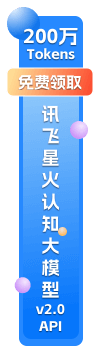API接口，开发服务，免费咨询服务

# 如何优雅而高效地使用Matplotlib实现数据可视化

## 关键点

Matplotlib 新手应该学习和使用面向对象的接口。

## 前提

• 1. 学习 Matplotlib 的基本术语，具体来说就是什么是 Figure 和 Axes。
• 2. 一直使用面向对象的界面，养成习惯。
• 3. 用基础的 pandas 绘图开始可视化。
• 4. 使用 seaborn 进行稍微复杂的数据可视化。
• 5. 使用 Matplotlib 自定义 pandas 或 seaborn 可视化。## 开始

### 开始，我打算设置输入，读取一些数据：

``````import pandas as pd
import matplotlib.pyplot as plt
from matplotlib.ticker import FuncFormatter``````top_10 = (df.groupby('name')['ext price', 'quantity'].agg({'ext price': 'sum', 'quantity': 'count'})
.sort_values(by='ext price', ascending=False))[:10].reset_index()
top_10.rename(columns={'name': 'Name', 'ext price': 'Sales', 'quantity': 'Purchases'}, inplace=True)``````

### 下图是数据。``````plt.style.available
['seaborn-dark',
'seaborn-dark-palette',
'fivethirtyeight',
'seaborn-whitegrid',
'seaborn-darkgrid',
'seaborn',
'bmh',
'classic',
'seaborn-colorblind',
'seaborn-muted',
'seaborn-white',
'seaborn-talk',
'grayscale',
'dark_background',
'seaborn-deep',
'seaborn-bright',
'ggplot',
'seaborn-paper',
'seaborn-notebook',
'seaborn-poster',
'seaborn-ticks',
'seaborn-pastel']``````

``plt.style.use('ggplot')``

``top_10.plot(kind='barh', y="Sales", x="Name")``## 自定义图表

``````fig, ax = plt.subplots()
top_10.plot(kind='barh', y="Sales", x="Name", ax=ax)``````

### 假设我们想调整一些轴标签，且 ax 变量中有多个轴，可以进行一些操作：

``````fig, ax = plt.subplots()
top_10.plot(kind='barh', y="Sales", x="Name", ax=ax)
ax.set_xlim([-10000, 140000])
ax.set_xlabel('Total Revenue')
ax.set_ylabel('Customer');````````````fig, ax = plt.subplots()
top_10.plot(kind='barh', y="Sales", x="Name", ax=ax)
ax.set_xlim([-10000, 140000])
ax.set(title='2014 Revenue', xlabel='Total Revenue', ylabel='Customer')````````````fig, ax = plt.subplots(figsize=(5, 6))
top_10.plot(kind='barh', y="Sales", x="Name", ax=ax)
ax.set_xlim([-10000, 140000])
ax.set(title='2014 Revenue', xlabel='Total Revenue')
ax.legend().set_visible(False)````````````def currency(x, pos):
'The two args are the value and tick position'
if x >= 1000000:
return '\${:1.1f}M'.format(x*1e-6)
return '\${:1.0f}K'.format(x*1e-3)``````

``````fig, ax = plt.subplots()
top_10.plot(kind='barh', y="Sales", x="Name", ax=ax)
ax.set_xlim([-10000, 140000])
ax.set(title='2014 Revenue', xlabel='Total Revenue', ylabel='Customer')
formatter = FuncFormatter(currency)
ax.xaxis.set_major_formatter(formatter)
ax.legend().set_visible(False)````````````# Create the figure and the axes
fig, ax = plt.subplots()

# Plot the data and get the averaged
top_10.plot(kind='barh', y="Sales", x="Name", ax=ax)
avg = top_10['Sales'].mean()

# Set limits and labels
ax.set_xlim([-10000, 140000])
ax.set(title='2014 Revenue', xlabel='Total Revenue', ylabel='Customer')

# Add a line for the average
ax.axvline(x=avg, color='b', label='Average', linestyle='--', linewidth=1)

# Annotate the new customers
for cust in [3, 5, 8]:
ax.text(115000, cust, "New Customer")

# Format the currency
formatter = FuncFormatter(currency)
ax.xaxis.set_major_formatter(formatter)

# Hide the legend
ax.legend().set_visible(False)``````## 图表

``fig, (ax0, ax1) = plt.subplots(nrows=1, ncols=2, sharey=True, figsize=(7, 4))``

``````# Get the figure and the axes
fig, (ax0, ax1) = plt.subplots(nrows=1,ncols=2, sharey=True, figsize=(7, 4))
top_10.plot(kind='barh', y="Sales", x="Name", ax=ax0)
ax0.set_xlim([-10000, 140000])
ax0.set(title='Revenue', xlabel='Total Revenue', ylabel='Customers')

# Plot the average as a vertical line
avg = top_10['Sales'].mean()
ax0.axvline(x=avg, color='b', label='Average', linestyle='--', linewidth=1)

# Repeat for the unit plot
top_10.plot(kind='barh', y="Purchases", x="Name", ax=ax1)
avg = top_10['Purchases'].mean()
ax1.set(title='Units', xlabel='Total Units', ylabel='')
ax1.axvline(x=avg, color='b', label='Average', linestyle='--', linewidth=1)

# Title the figure
fig.suptitle('2014 Sales Analysis', fontsize=14, fontweight='bold');

# Hide the legends
ax1.legend().set_visible(False)
ax0.legend().set_visible(False)``````Matplotlib 支持多种不同文件保存格式。你可以使用 fig.canvas.get_supported_filetypes() 查看系统支持的文件格式：

``````fig.canvas.get_supported_filetypes()
{'eps': 'Encapsulated Postscript',
'jpeg': 'Joint Photographic Experts Group',
'jpg': 'Joint Photographic Experts Group',
'pdf': 'Portable Document Format',
'pgf': 'PGF code for LaTeX',
'png': 'Portable Network Graphics',
'ps': 'Postscript',
'raw': 'Raw RGBA bitmap',
'rgba': 'Raw RGBA bitmap',
'svg': 'Scalable Vector Graphics',
'svgz': 'Scalable Vector Graphics',
'tif': 'Tagged Image File Format',
'tiff': 'Tagged Image File Format'}``````

``fig.savefig('sales.png', transparent=False, dpi=80, bbox_inches="tight")``

## 结论API资讯

API接口，开发服务，免费咨询服务

## 关键点

Matplotlib 新手应该学习和使用面向对象的接口。

## 前提

• 1. 学习 Matplotlib 的基本术语，具体来说就是什么是 Figure 和 Axes。
• 2. 一直使用面向对象的界面，养成习惯。
• 3. 用基础的 pandas 绘图开始可视化。
• 4. 使用 seaborn 进行稍微复杂的数据可视化。
• 5. 使用 Matplotlib 自定义 pandas 或 seaborn 可视化。## 开始

### 开始，我打算设置输入，读取一些数据：

``````import pandas as pd
import matplotlib.pyplot as plt
from matplotlib.ticker import FuncFormatter``````top_10 = (df.groupby('name')['ext price', 'quantity'].agg({'ext price': 'sum', 'quantity': 'count'})
.sort_values(by='ext price', ascending=False))[:10].reset_index()
top_10.rename(columns={'name': 'Name', 'ext price': 'Sales', 'quantity': 'Purchases'}, inplace=True)``````

### 下图是数据。``````plt.style.available
['seaborn-dark',
'seaborn-dark-palette',
'fivethirtyeight',
'seaborn-whitegrid',
'seaborn-darkgrid',
'seaborn',
'bmh',
'classic',
'seaborn-colorblind',
'seaborn-muted',
'seaborn-white',
'seaborn-talk',
'grayscale',
'dark_background',
'seaborn-deep',
'seaborn-bright',
'ggplot',
'seaborn-paper',
'seaborn-notebook',
'seaborn-poster',
'seaborn-ticks',
'seaborn-pastel']``````

``plt.style.use('ggplot')``

``top_10.plot(kind='barh', y="Sales", x="Name")``## 自定义图表

``````fig, ax = plt.subplots()
top_10.plot(kind='barh', y="Sales", x="Name", ax=ax)``````

### 假设我们想调整一些轴标签，且 ax 变量中有多个轴，可以进行一些操作：

``````fig, ax = plt.subplots()
top_10.plot(kind='barh', y="Sales", x="Name", ax=ax)
ax.set_xlim([-10000, 140000])
ax.set_xlabel('Total Revenue')
ax.set_ylabel('Customer');````````````fig, ax = plt.subplots()
top_10.plot(kind='barh', y="Sales", x="Name", ax=ax)
ax.set_xlim([-10000, 140000])
ax.set(title='2014 Revenue', xlabel='Total Revenue', ylabel='Customer')````````````fig, ax = plt.subplots(figsize=(5, 6))
top_10.plot(kind='barh', y="Sales", x="Name", ax=ax)
ax.set_xlim([-10000, 140000])
ax.set(title='2014 Revenue', xlabel='Total Revenue')
ax.legend().set_visible(False)````````````def currency(x, pos):
'The two args are the value and tick position'
if x >= 1000000:
return '\${:1.1f}M'.format(x*1e-6)
return '\${:1.0f}K'.format(x*1e-3)``````

``````fig, ax = plt.subplots()
top_10.plot(kind='barh', y="Sales", x="Name", ax=ax)
ax.set_xlim([-10000, 140000])
ax.set(title='2014 Revenue', xlabel='Total Revenue', ylabel='Customer')
formatter = FuncFormatter(currency)
ax.xaxis.set_major_formatter(formatter)
ax.legend().set_visible(False)````````````# Create the figure and the axes
fig, ax = plt.subplots()

# Plot the data and get the averaged
top_10.plot(kind='barh', y="Sales", x="Name", ax=ax)
avg = top_10['Sales'].mean()

# Set limits and labels
ax.set_xlim([-10000, 140000])
ax.set(title='2014 Revenue', xlabel='Total Revenue', ylabel='Customer')

# Add a line for the average
ax.axvline(x=avg, color='b', label='Average', linestyle='--', linewidth=1)

# Annotate the new customers
for cust in [3, 5, 8]:
ax.text(115000, cust, "New Customer")

# Format the currency
formatter = FuncFormatter(currency)
ax.xaxis.set_major_formatter(formatter)

# Hide the legend
ax.legend().set_visible(False)``````## 图表

``fig, (ax0, ax1) = plt.subplots(nrows=1, ncols=2, sharey=True, figsize=(7, 4))``

``````# Get the figure and the axes
fig, (ax0, ax1) = plt.subplots(nrows=1,ncols=2, sharey=True, figsize=(7, 4))
top_10.plot(kind='barh', y="Sales", x="Name", ax=ax0)
ax0.set_xlim([-10000, 140000])
ax0.set(title='Revenue', xlabel='Total Revenue', ylabel='Customers')

# Plot the average as a vertical line
avg = top_10['Sales'].mean()
ax0.axvline(x=avg, color='b', label='Average', linestyle='--', linewidth=1)

# Repeat for the unit plot
top_10.plot(kind='barh', y="Purchases", x="Name", ax=ax1)
avg = top_10['Purchases'].mean()
ax1.set(title='Units', xlabel='Total Units', ylabel='')
ax1.axvline(x=avg, color='b', label='Average', linestyle='--', linewidth=1)

# Title the figure
fig.suptitle('2014 Sales Analysis', fontsize=14, fontweight='bold');

# Hide the legends
ax1.legend().set_visible(False)
ax0.legend().set_visible(False)``````Matplotlib 支持多种不同文件保存格式。你可以使用 fig.canvas.get_supported_filetypes() 查看系统支持的文件格式：

``````fig.canvas.get_supported_filetypes()
{'eps': 'Encapsulated Postscript',
'jpeg': 'Joint Photographic Experts Group',
'jpg': 'Joint Photographic Experts Group',
'pdf': 'Portable Document Format',
'pgf': 'PGF code for LaTeX',
'png': 'Portable Network Graphics',
'ps': 'Postscript',
'raw': 'Raw RGBA bitmap',
'rgba': 'Raw RGBA bitmap',
'svg': 'Scalable Vector Graphics',
'svgz': 'Scalable Vector Graphics',
'tif': 'Tagged Image File Format',
'tiff': 'Tagged Image File Format'}``````

``fig.savefig('sales.png', transparent=False, dpi=80, bbox_inches="tight")``

## 结论• 短信API服务
• 银行卡四元素检测[简]
• 身份证实名认证
• 手机状态查询
• 三网手机实名制认证[简]
• 身份证OCR识别
• 证件识别
• 企业工商信息

• 短信API服务
• 银行卡四元素检测[简]
• 身份证实名认证
• 手机状态查询
• 三网手机实名制认证[简]
• 身份证OCR识别
• 证件识别
• 企业工商信息
• 确定×0512-88869195

Data Drives The Future